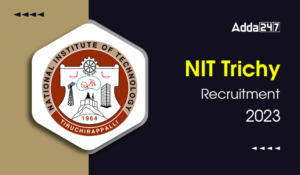Engineering Jobs   »   Mechincal Engineering quizs   »   UPPSC Lecturer Recruitment 2021, UPPSC Lecturer...

# UPPSC Lec’21 ME: Daily Practices Quiz. 16-Dec-2021

Quiz: Mechanical Engineering
Exam: UPPSC AE/Lecturer
Topic: Miscellaneous

Each question carries 3 marks
Negative marking: 1 mark
Time: 8 Minutes

Q1. Match List-I (Process index ‘n’) with List-II (T-s traces) and select the correct answer using the code given below the lists
List I
A. 0
B. 1.0
C. 1.4
D. ∞
List IICodes
A B C d
(a) 3 1 4 2
(b) 2 1 4 3
(c) 3 4 1 2
(d) 2 4 1 3

Q2. Match List-I with List-II and select the correct answer using the codes given below the lists:
List I
Work done in a polytropic process
Work done in steady flow process
Heat transfer in a reversible adiabatic process
Work done in an isentropic process
List- II
-∫▒Vdp
Zero
(p_1 V_1-p_2 V_2)/(γ-1)
4. (p_1 V_1-p_2 V_2)/(n-1)
Codes
A B C D
(a) 4 1 3 2
(b) 1 4 2 3
(c) 4 1 2 3
(d) 1 2 3 4

Q3. In an elliptic trammel, the length of the link connecting the two sliders is 100 mm. The tracing pen is placed on 150 mm extension of this link. The major and minor axes of the ellipse traced by the mechanism would be
(a) 250 mm and 150 mm
(b) 250 mm and 100 mm
(c) 500 mm and 300 mm
(d) 500 mm and 200 mm

Q4. In a crank and slotted lever quick return motion mechanism, the distance between the fixed centres is 160 mm and the driving crank is 80 mm long. The ratio of time taken by cutting and return strokes is
(a) 0.5
(b) 1
(c) 1.5
(d) 2

Q5. The problem of interference in involute profile gears can be overcome by which one of the following means?
(a) Decreasing the centre distance
(b) Using composite profile with cycloidal curve at the root of the tooth
(c) Using sub teeth of height more than the full depth teeth
(d) Proper lubrication

Q6. The flywheel of a steam engine has a radius of gyration of 1 m and mass 2000 kg. The starting torque of the engine is 2000 N-m. The kinetic energy of the flywheel after 10 seconds from start is
(a) 75 kN-m
(b) 100 kN-m
(c) 125 kN-m
(d) 150 kN-m

Solutions

S1. Ans.(c)
Sol.S2. Ans.(c)
Sol.
A. Work done in a polytropic process: (p_1 V_1-p_2 V_2)/(n-1)
B. Work done in steady flow process -∫▒Vdp
C. Heat transfer in a reversible adiabatic process: zero
D. Work done is an isentropic process: (p_1 V_1-p_2 V_2)/(γ-1)

S3. Ans.(c)
Sol.Semi major axis
= AC = AB + BC = 250 mm
Semi minor axis
= BC = 150 mm
Major axis = 250 × 2 = 500 mm
Minor axis = 150 × 2 = 300 mm

S4. Ans.(d)
Sol.cos β/2=80/160=1/2
β=120°
θ=240°
Hence, θ/β=(Time taken by cutting stroke)/(Time taken by return stroke)
=240/120=2

S5. Ans.(b)
Sol.

S6. Ans.(b)
Sol.
I = mk² = 2000 × (1)²= 2000
T= 2000 Nm
T = Iα
2000 = 2000 × α
After 10 sec,
ω = ω0 + at = 0t + 1 × 10 = 10 rad/s
E=1/2 Iω^2=1/2×2000×10
=10^5=100 kNm

Sharing is caring!

•GATE 2023 Admit Card Out, Download Link ...
•OSSC JE Admit Card 2023, Direct Link to ...
•BARC OCES Apply Online 2023, Online Appl...
•BSF Recruitment 2023 Notification Out, A...
•OMC Recruitment 2023, Notification Out F...
•NIT Trichy Recruitment 2023, Apply Now F...
•SECL Recruitment 2023 Notification Out F...